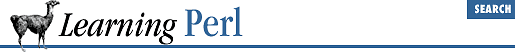home | O'Reilly's CD bookshelfs | FreeBSD | Linux | Cisco | Cisco Exam

### A.14 Chapter 15, Other Data Transformation

1. Here's one way to do it:

```while (

<>) {
chomp;
\$slash =

rindex(\$_,"/");
if (\$slash > -1) {

substr(\$_,0,\$slash);
\$tail = substr(\$_,\$slash+1);
} else {
}
}```

Each line read by the diamond operator is first chomped (tossing the newline). Next we look for the rightmost slash in the line, using ``` rindex()``` . The next two lines break the string apart using ``` substr()``` . If there's no slash, the result of the ``` rindex``` is ``` -1``` , so we hack around that. The final line within the loop prints the results.

2. Here's one way to do it:

```chomp(@nums = <STDIN>); # note special use of chomp
@nums =

sort { \$a <=> \$b } @nums;
foreach (@nums) {
printf "%30g\n", \$_;
}```

The first line grabs all of the numbers into the ``` @nums``` array. The second line sorts the array numerically, using an inline definition for a sorting order. The ``` foreach``` loop prints the results.

3. Here's one way to do it:

```open(PW,"/etc/passwd") || die "How did you get logged in?";
while (<PW>) {
chomp;
(\$user, \$gcos) = (split /:/)[0,4];
(\$real) = split(/,/, \$gcos);
\$real{\$user} = \$real;
(\$last) = (split /\s+/, \$real)[-1];
\$last{\$user} = "\L\$last";
}
close(PW);

for (

sort by_last keys %last) {
printf "%30s %8s\n", \$real{\$_}, \$_;
}

sub by_last { (\$last{\$a}

cmp \$last{\$b}) || (\$a cmp \$b) }```

The first loop creates ``` %last``` hash, consisting of login names for keys and user's last names for the corresponding values, and the ``` %real``` hash, containing the full real names instead. The last names are all converted to lowercase, so that FLINTSTONE, Flintstone, and flintstone all sort near each other.

The second loop prints ``` %real``` out, ordered by the values of ``` %last``` , using the sort definition presented in ``` by_last``` subroutine.

4. Here's one way to do it:

```

while (<>) {

substr(\$_,0,1) =~ tr/a-z/A-Z/;
substr(\$_,1) =~ tr/A-Z/a-z/;
print;
}```

For each line read by the diamond operator, we use two ``` tr``` operators, each on a different portion of the string. The first ``` tr``` operator uppercases the first character of the line, and the second ``` tr``` operator lowercases the remainder. The result is printed.

Here's another way to do this, using only double-quoted string operators:

```while (<>) {
print "\u\L\$_";
}```

Give yourself an extra five points if you thought of that instead.A.13 Chapter 14, Process ManagementA.15 Chapter 16, System Database Access